Willmore functional

The Willmore functional of an immersed surface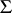into the Euclidean spaceis defined bywhere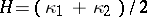is the mean curvature of the surface. Here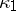,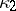are the two classical principal curvatures of the surface (cf. also Principal curvature) and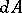is the area element of the induced metric on. Moreover, it is assumed that the integralconverges, which is guaranteed ifis compact, as is usually assumed. Critical points of the functionalare called Willmore surfaces and are characterized by the Euler equation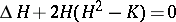corresponding to the variational problem(cf. also Variation of a functional; Variational calculus; Variational problem). Here,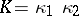is the Gaussian curvature of the surface andis its Laplace–Beltrami operator (cf. Laplace–Beltrami equation). An alternative functional tois the functional given byBecause of the Gauss–Bonnet theorem, ifis assumed to be compact and without boundary, then, where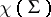denotes the Euler characteristic of the surface, so thatand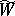have the same critical points.

The functionalwas first studied by W. Blaschke (1929) and G. Thomsen (1923), who established the most important property of: The functionalis invariant under conformal changes of metric of the ambient space. They considered it as a substitute for the area of surfaces in conformal geometry. For that reason, Willmore surfaces were called Konformminimalflächen (conformally minimal surfaces; cf. also Minimal surface).

These results were forgotten for some time and were rediscovered by T.J. Willmore in 1965, reopening interest in the subject. He proved that for any compact orientable surfaceimmersed in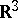one has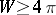, equality holding if and only ifis embedded as a round sphere. In an attempt to improve this inequality for surfaces of higher genus, Willmore also showed that for anchor rings, obtained by rotating a circle of radiusabout an axis in its plane at distance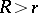from its centre, it holds that, equality holding when. For various reasons, Willmore also conjectured that any torus immersed insatisfies the inequality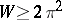. This inequality is known as the Willmore conjecture.

Although the general case remains an open problem (as of 2000), the Willmore conjecture has been proved for various special classes of tori. For instance, it is known to be true for a torus embedded inas a tube of constant circular cross-section (K. Shiohama and R. Takagi, 1970, and Willmore, 1971) as well as for tori of revolution (J. Langer and D. Singer, 1984). In 1982, P. Li and S.T. Yau showed that the Willmore conjecture is true for conformal structures near that of the special-torus. The set of conformal structures for which the Willmore conjecture is true was enlarged by S. Montiel and A. Ros (1985). Recently (2000), B. Ammann proved it under the condition that the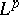-norm of the Gaussian curvature is sufficiently small.

On the other hand, in 1978 J.L. Weiner generalized the Willmore functional by considering immersions of an orientable surface, with or without boundary, into a Riemannian manifold of constant sectional curvature. Instead ofhe considered the integralwhere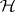denotes the mean curvature vector field of the surface, and obtained the corresponding Euler equation. In the particular case when the ambient space is the unit sphere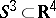, the Euler equation becomes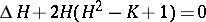, so that every minimal surface in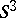is a Willmore surface. An interesting consequence of Weiner's result is the proof that stereographic projections of compact minimal surfaces inproduce Willmore surfaces in. For instance, the special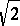-torus infor which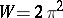corresponds to the stereographic projection of the Clifford torus, embedded as a minimal surface in. Moreover, H.B. Lawson proved in 1970 that every compact, orientable surface can be minimally embedded in; it follows from this that there are embedded Willmore surfaces inof arbitrary genus (cf. also Genus of a surface). On the other hand, Weiner also considered questions of stability of Willmore surfaces by considering the second variation of the Willmore functional. In particular, he showed that the special minimizing-torus is stable.

Most of the known examples of embedded Willmore surfaces income from compact minimal surfaces in the unit sphere. In 1985, U. Pinkall found the first examples of compact embedded Willmore surfaces that are not stereographic projections of compact embedded minimal surfaces in. Using results of Langer and Singer on elastic curves on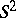, he exhibited an infinite series of embedded Willmore tori inthat cannot be obtained by stereographic projection of minimal surfaces in. All of these tori are, however, unstable critical points ofand hence are not candidates for absolute minima.

Important contributions to the study of Willmore surfaces are made by R.L. Bryant, who classified all Willmore immersions of a topological sphere intoand determined the critical values of the Willmore functional; Li and Yau, who introduced the concept of conformal volume; M. Gromov, who introduced the concept of visual volume, closely related to the conformal volume; and others.

Finally, it is worth pointing out the relation between the Willmore functional and quantum physics, including the theory of liquid membranes, two-dimensional gravity and string theory. For instance, in string theory the functionalis known as the Polyakov extrinsic action and in membrane theory it is the Helfrich free energy.